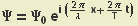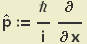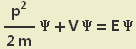## Friday, 1 June 2007

### Schrödinger equation

The Schrödinger equation is be pretty basic stuff but it is pretty cool. This is how it comes from the energy conservation law:

We start out with de Broglie (and the theory of relativity)and we know that all particles are also waves, a typical wave equation beingJust for the fun of it we make the derivativeand we find out that we can get the momentum through deriving the function. Who would have thought that?

Next we define the momentum operatorand we assume that for every possible wave function the following is true (this and the definition of the position operator are the axioms of quantum mechanics):With this knowledge we can look at the energy conservation law of mechanics (T ... kinetic energy, V ... potential energy, E ... total energy)The kinetic energy can be rewrittenWe can multiply this equation by ΨFinally we replace the momentum by the momentum operatorAnonymous said...

Isn't the momentum operator [h/(2πi)](d/dx) = -ihbar(d/dx)

Felix said...

thanks for the correction, I fixed it. I don't know why I moved the 2π from the numerator into the denominator in the third equation.Anonymous said...

Schrödinger equation is blogged!
That's pretty cool!
Recently I've made a QM post too:

Are Orbitals Real?!

Would appreciate your opinion on that.